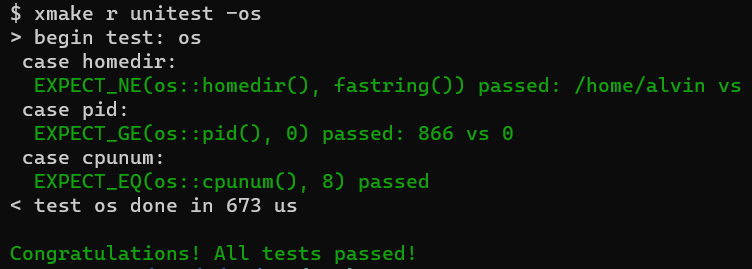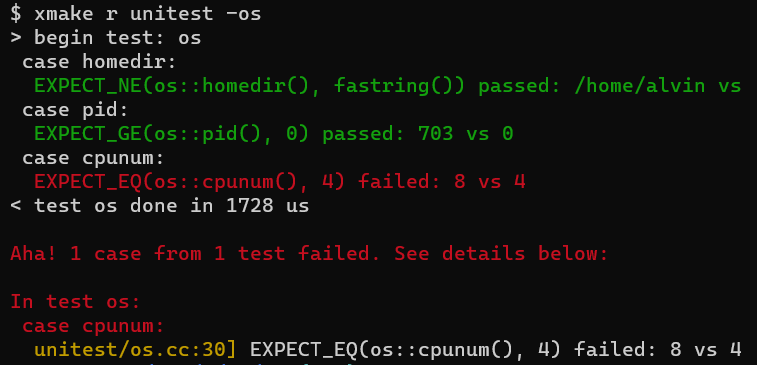Unitest

include: co/unitest.h.

## #Basic concepts

co.unitest is a unit testing framework, similar to google gtest, but easier to use.

### #Test Units and Test Cases

A test program can be divided into multiple test units according to functions or modules, and there can be multiple test cases under each test unit. For example, a test unit can be defined for a class (or module) in C++, and a test case can be defined for each method in the class (or module).

``````DEF_test(test_name) {
DEF_case(a) {
// write test code here
}

DEF_case(b) {
// write test code here
}
}
``````

In the above example, `DEF_test` defines a test unit (actually defines a function), and `DEF_case` defines the test case, a test case is actually a code block in the function.

### #DEF_test

``````#define DEF_test(_name_) \
DEF_bool(_name_, false, "enable this test if true"); \
... \
void _co_ut_##_name_(unitest::xx::Test& _t_)
``````
• The `DEF_test` macro is used to define a test unit, and the parameter `_name_` is the name of the test unit.
• The first line of the macro defines a bool flag, which is the switch of the test unit. For example, `DEF_test(os)` defines a test unit os, and we can use `-os` in command line to enable test cases in this unit.
• The last line of the macro defines the function corresponding to the test unit.

### #DEF_case

``````#define DEF_case(name) \
_t_.c = #name; \
cout << " case " << #name << ':' << endl;
``````
• The `DEF_case` macro is used to define a test case in the test unit. The parameter `name` is the name of the test case. It must be used inside the function defined by `DEF_test`.
• The name of a test unit must be albe to use as part of the class name or variable name. The test case name does not have this restriction. For example, `DEF_case(sched.Copool)` is also reasonable.
• The code of the test case is generally enclosed by a pair of curly braces to isolate it from other test cases.
• DEF_test may not contain any DEF_case. In this case, `co.unitest` will create a default test case.

### #EXPECT assertion

``````#define EXPECT(x) ...
#define EXPECT_EQ(x, y) EXPECT_OP(x, y, ==, "EQ")
#define EXPECT_NE(x, y) EXPECT_OP(x, y, !=, "NE")
#define EXPECT_GE(x, y) EXPECT_OP(x, y, >=, "GE")
#define EXPECT_LE(x, y) EXPECT_OP(x, y, <=, "LE")
#define EXPECT_GT(x, y) EXPECT_OP(x, y, >, "GT")
#define EXPECT_LT(x, y) EXPECT_OP(x, y, <, "LT")
``````
• `EXPECT` asserts that `x` is true, and x can be any expression with a value of type bool.
• `EXPECT_EQ` asserts `x == y`.
• `EXPECT_NE` asserts `x != y`.
• `EXPECT_GE` asserts that `x >= y`.
• `EXPECT_LE` asserts that `x <= y`.
• `EXPECT_GT` asserts that `x > y`.
• `EXPECT_LT` asserts that `x < y`.
• When defining a test case with `DEF_case`, you can use these macro assertions. If an assertion fails, it means that the test case fails. The terminal will print related error messages in red color.

## #Write test code

### #Test code example

``````#include "co/unitest.h"
#include "co/os.h"

DEF_test(os) {
DEF_case(homedir) {
EXPECT_NE(os::homedir(), "");
}

DEF_case(pid) {
EXPECT_GE(os::pid(), 0);
}

DEF_case(cpunum) {
EXPECT_GT(os::cpunum(), 0);
}
}

int main(int argc, char** argv) {
flag::parse(argc, argv);
unitest::run_tests();
return 0;
}
``````
• The above code defines a test unit named os, and os has 3 test cases.
• When running the test program, you can use `-os` in the command line to enable this unit test.
• In the main function, you need call `flag::parse()` to parse the command line parameters, and then call the `run_tests()` method provided by `co.unitest` to run the unit test code.

### #Default test case

``````DEF_test(os) {
EXPECT_NE(os::homedir(), "");
EXPECT_GE(os::pid(), 0);
EXPECT_GT(os::cpunum(), 0);
}
``````
• The above code does not contain any DEF_case, `co.unitest` will create a default test case named `default`.
• For more complex test codes, it is generally not recommended to use the default test cases. It is better to divide them into different cases so that the code looks clearer.

## #Build and run the test program

### #Build the unitest code

``````xmake -b unitest
``````
• Run the above command in the root directory of coost to compile the unit test code in co/unitest and generate the `unitest` binary program .

### #Run all test cases

``````xmake r unitest
``````
• Run all test cases by default.

### #Run test cases in specified test units

``````# Run only test cases in the os test unit
xmake r unitest -os

# Run test cases in the os or json test units
xmake r unitest -os -json
``````

### #Test result example

• All tests passed• Test case failed•English